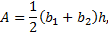## Saturday, December 31, 2011

### Field Measurement of Quadrilaterals Using Only a Tape Measure

##### Trapezoids
A common nonrectangular area that needs to me measured in the field is a trapezoid. Sometimes shapes are approximated by a trapezoid, if a rectangle is not considered a reasonable approximation or not easily visualized. The well-known formula for the area of a trapezoid iswhere b1 and b2 are the lengths of the two parallel sides and h is the distance between them (measured perpendicularly to the parallel sides, of course). It is noteworthy that the (b1+b2)/2 can be interpreted as the average of the lengths of the parallel sides. This is the length of a line referred to as the median of the trapezoid. In the field, it is often more convenient/faster to measure the median and the height (h, also called the altitude) than to measure both bases and the height. If the location of the median can be “eye-balled” with sufficient precision for the purposes being met, this may increase productivity by decreasing the number of measurements needed. For more information on the trapezoid and the median, see a description here.
##### General Case Quadrilateral
There are (at least) two approaches to measuring quadrilaterals when nothing is known about the internal angles or “parallelness” of nonadjacent lines. The following diagram displays both methods.

We can determine the area of this shape by making 5 measurements and produce an exact picture, or by taking 3 measurements and getting an approximate area. Both are “area by triangles” methods.
##### Area by Triangles – Heron Method
If we measure the sides AB, BC, CD, DA, and one of the diagonals (either AC or BD), we can use Heron’s theorem to determine the area of the triangles on either side of the chosen diagonal. We can use the law of cosines and law of sines to determine the angles. Alternatively, a basic AutoCAD drawing using temporary construction-line circles would allow you to determine these results without manual calculations. This would give us a full description of the area. This method also generalizes well to an arbitrary number of sides.
##### Area by Triangles – By Altitude
On the other hand, we could measure the length of a diagonal (say, AC), leave the measuring tape (or a string line) in place and use a second tape measure to measure from the other vertices (B and D) to the diagonal. As long as the accuracy/precision of the result desired is not too high, we can “eye-ball” perpendicular to the diagonal to get the heights of the triangles to an acceptable accuracy. The area calculation is obvious from there. This method involves fewer measurements, minimal extra equipment, and is quicker to calculate, which may make it more efficient for a quadrilateral. It does not generalize to an n-sided figure as easily as the previous method, but could be done with additional string lines used simultaneously.